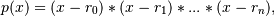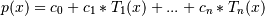# numpy.polynomial.chebyshev.chebfromroots¶

`numpy.polynomial.chebyshev.``chebfromroots`(roots)[source]

Generate a Chebyshev series with given roots.

The function returns the coefficients of the polynomialin Chebyshev form, where the r_n are the roots specified in roots. If a zero has multiplicity n, then it must appear in roots n times. For instance, if 2 is a root of multiplicity three and 3 is a root of multiplicity 2, then roots looks something like [2, 2, 2, 3, 3]. The roots can appear in any order.

If the returned coefficients are c, thenThe coefficient of the last term is not generally 1 for monic polynomials in Chebyshev form.

Parameters: roots : array_like Sequence containing the roots. out : ndarray 1-D array of coefficients. If all roots are real then out is a real array, if some of the roots are complex, then out is complex even if all the coefficients in the result are real (see Examples below).

`polyfromroots`, `legfromroots`, `lagfromroots`, `hermfromroots`, `hermefromroots.`

Examples

```>>> import numpy.polynomial.chebyshev as C
>>> C.chebfromroots((-1,0,1)) # x^3 - x relative to the standard basis
array([ 0.  , -0.25,  0.  ,  0.25])
>>> j = complex(0,1)
>>> C.chebfromroots((-j,j)) # x^2 + 1 relative to the standard basis
array([ 1.5+0.j,  0.0+0.j,  0.5+0.j])
```

#### Previous topic

numpy.polynomial.chebyshev.chebroots

#### Next topic

numpy.polynomial.chebyshev.chebfit